NCERT Solutions for Class 8 Maths Chapter 6 Squares and Square Roots Ex 6.1 are part of NCERT Solutions for Class 8 Maths. Here we have given NCERT Solutions for Class 8 Maths Chapter 6 Squares and Square Roots Ex 6.1.

 Board CBSE Textbook NCERT Class Class 8 Subject Maths Chapter Chapter 6 Chapter Name Squares and Square Roots Exercise Ex 6.1 Number of Questions Solved 9 Category NCERT Solutions

## NCERT Solutions for Class 8 Maths Chapter 6 Squares and Square Roots Ex 6.1

Question 1.
What will be the unit digit of the squares of the following numbers ?
(i) 81
(ii) 272
(iii) 799
(iv) 3853
(v) 1234
(vi) 26387
(vii) 52698
(viii) 99880
(ix) 12796
(x) 55555
Solution.
(i) 81
∵ $$1\times 1$$
∴ The unit digit of the square of the number 81 will be 1.

(ii) 272
∵ $$2\times 2$$
∴ The unit digit of the square of the number 272 will be 4.

(iii) 799
∵ $$9\times 9$$
∴ The unit digit of the square of the number 799 will be 1.

(iv) 3853
∵ $$3\times 3$$
∴ The unit digit of the square of the number 3853 will be 9

(v) 1234
∵ $$4\times 4$$
∴ The unit digit of the square of the number 1234 will be 6.

(vi) 26387
∵ $$7\times 7$$
∴ The unit digit of the square of the number 26387 will be 9.

(vii) 52698
∵ $$8\times 8$$
∴ The unit digit of the square of the number 52698 will be 4.

(viii) 99880
∵ $$0\times 0$$
∴ The unit digit of the square of the number 99880 will be 0.

(ix) 12796
∵ $$6\times 6$$
∴ The unit digit of the square of the number 12796 will be 6.

(x) 55555
∵ $$5\times 5$$
∴ The unit digit of the square of the number 55555 will be 5.

Question 2.
The following numbers are obviously not perfect squares. Give reason.
(i) 1057
(ii) 23453
(iii) 7928
(iv) 222222
(v) 64000
(vi) 89722
(vii) 222000
(viii) 505050.
Solution.
(i) 1057
The number 1057 is not a perfect square because it ends with 7 at unit’s place whereas the square numbers end with 0, 1, 4, 5, 6 or 9 at unit’s place.

(ii) 23453
The number 23453 is not a perfect square because it ends with 3 at unit’s place whereas the square numbers end with 0, 1, 4, 5, 6 or 9 at unit’s place.

(iii) 7928
The number 7928 is not a perfect square because it ends with 8 at unit’s place whereas the square numbers end with 0, 1, 4, 5, 6 or 9 at unit’s place.

(iv) 222222
The number 222222 is not a perfect square because it ends with 2 at unit’s place whereas the Square numbers end with 0, 1, 4, 5, 6 or 9 at unit’s place.

(v) 64000
The number 64000 is not a square number because it has 3 (an odd number of) zeros at the end whereas the number of zeros at the end of a square number ending with zeros is always even.

(vi) 89722
The number 89722 is not a square number because it ends in 2 at unit’s place whereas the square numbers end with 0, 1, 4, 5, 6 or 9 at unit’s place.

(vii) 222000
The number 222000 is not a square number because it has 3 (an odd number of) zeros at the end whereas the number of zeros at the end of a square number ending with zeros is always even.

(viii) 505050
The number 505050 is not a square number because it has 1 (an odd number of) zeros at the end whereas the number of zeros at the end of a square number ending with zeros is always even.

Question 3.
The squares of which of the following would be odd numbers ?
(i) 431
(ii) 2826
(iii) 7779
(v) 82004.
Solution.
(i) 431
∵ 431 is an odd number
∴ Its square will also be an odd number.

(ii) 2826
∵ 2826 is an even number
∴ Its square will not be an odd number.

(iii) 7779
∵ 7779 is an odd number
∴ Its square will be an odd number.

(iv) 82004
∵ 82004 is an even number
∴ Its square will not be an odd number.

Question 4.
Observe the following pattern and find the missing digits: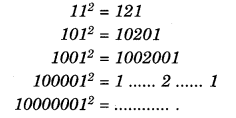Solution.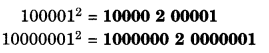Question 5.
Observe the following pattern and supply the missing numbers:Solution.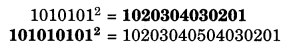Question 6.
Using the given pattern, find the missing numbers: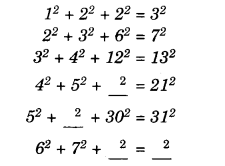Solution.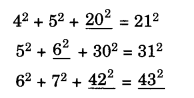Question 7.
(i) 1 + 3 + 5+7 + 9
(ii) 1 + 3 + 5 + 7 + 9 + 11 + 13 + 15 +17 + 19
(iii) 1+3 + 5 + 7 + 9 + 11 + 13 + 15 + 17 + 19 + 21 +23.
Solution.
(i)
l + 3 + 5 + 7 + 9 = sum of first five odd natural numbers = $${ 5 }^{ 2 }$$ = 25

(ii)
1 + 3 + 5 + 7 + 9 + 11 + 13 + 15 + 17 + 19 = sum of first ten odd natural numbers = $${ 10 }^{ 2 }$$ = 100

(iii)
1 + 3 + 5 + 7 + 9 + 11 + 13 + 15 + 17 + 19 + 21 + 23 = sum of first twelve odd natural numbers = $${ 12 }^{ 2 }$$= 144.

Question 8.
(i) Express 49 as the sum of 7 odd numbers.
(ii) Express 121 as the sum of 11 odd numbers.
Solution.
(i)
49 (= $${ 7 }^{ 2 }$$)
= 1 + 3 + 5 + 7 + 9 + 11 + 13

(ii)
121 (= $${ 11 }^{ 2 }$$)
= 1 + 3 + 5 + 7 + 9 + 11 + 13 + 15 + 17 + 19 + 21.

Question 9.
How many numbers lie between squares of the following numbers ?
(i) 12 and 13
(ii) 25 and 26
(iii) 99 and 100.
Solution.
(i) 12 and 13
Here, n = 12
∴ 2n = 2 x 12 = 24
So, 24 numbers lie between squares of i the numbers 12 and 13.

(ii) 25 and 26
Here, n = 25
∴ 2n = 2 x 25 = 50
So, 50 numbers lie between squares of the numbers 25 and 26.

(iii) 99 and 100
Here, n = 99
∴ 2n = 2 x 99 = 198
So, 198 numbers lie between squares of of the numbers 99 and 100.

We hope the NCERT Solutions for Class 8 Maths Chapter 6 Squares and Square Roots Ex 6.1 are part help you. If you have any query regarding NCERT Solutions for Class 8 Maths Chapter 6 Squares and Square Roots Ex 6.1 are part, drop a comment below and we will get back to you at the earliest.